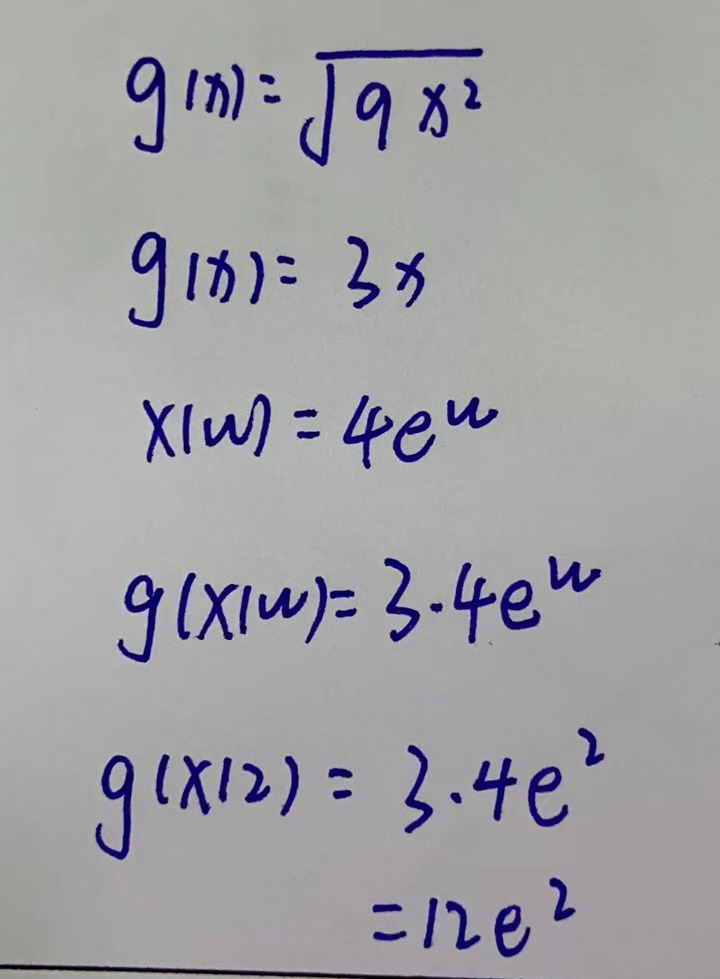### Still have math questions?

Algebra
Question.Rewrite the given pair of functions as one composite function. (Be sure to use $$w$$ as the independent variable.)

$$g ( x ) = \sqrt { 9 x ^ { 2 } } ; x ( w ) = 4 e ^ { w }$$

$$g ( x ) ) =$$

Evaluate the composite function at 2. (Round your answer to two decimal places.)

$$g ( x ( 2 ) ) =$$